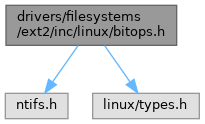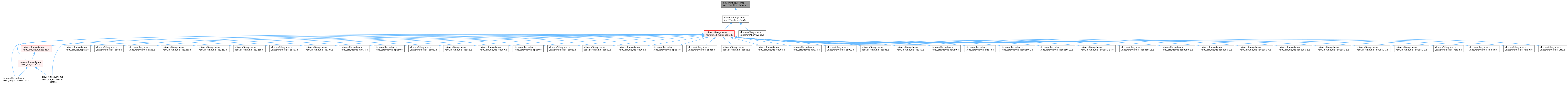ReactOS 0.4.15-dev-6680-g8c76870
bitops.h File Reference
`#include <ntifs.h>`
`#include <linux/types.h>`
Include dependency graph for bitops.h:This graph shows which files directly or indirectly include this file:Go to the source code of this file.

## Macros

#define ffz(x)   __ffs(~(x))

## Functions

int find_next_zero_bit (const unsigned long *addr, int size, int offset)

static unsigned long __ffs (unsigned long word)

static unsigned find_first_bit (const unsigned long *addr, unsigned size)

static int ffs (int x)

static int fls (int x)

static int fls64 (__u64 x)

static __inline int get_bitmask_order (unsigned int count)

static __inline int get_count_order (unsigned int count)

static __u32 rol32 (__u32 word, unsigned int shift)

static __u32 ror32 (__u32 word, unsigned int shift)

static unsigned fls_long (unsigned long l)

static unsigned long hweight32 (unsigned long w)

static unsigned long hweight64 (__u64 w)

static unsigned long hweight_long (unsigned long w)

## ◆ ffz

 #define ffz ( x ) __ffs(~(x))

find_next_bit - find the next set bit in a memory region @addr: The address to base the search on @offset: The bitnumber to start searching at @size: The maximum size to search

Definition at line 109 of file bitops.h.

## ◆ find_first_zero_bit

find_first_zero_bit - find the first zero bit in a memory region @addr: The address to start the search at @size: The maximum size to search

Returns the bit number of the first zero bit, not the number of the byte containing a bit.

Definition at line 28 of file bitops.h.

## ◆ for_each_bit

 #define for_each_bit ( bit, addr, size )
Value:
for ((bit) = find_first_bit((addr), (size)); \
(bit) < (size); \
(bit) = find_next_bit((addr), (size), (bit) + 1))
static unsigned find_first_bit(const unsigned long *addr, unsigned size)
Definition: bitops.h:83
GLsizeiptr size
Definition: glext.h:5919
Definition: glext.h:9621

Definition at line 194 of file bitops.h.

## ◆ __ffs()

 static unsigned long __ffs ( unsigned long word )
inlinestatic

__ffs - find first bit in word. @word: The word to search

Undefined if no bit exists, so code should check against 0 first.

Definition at line 44 of file bitops.h.

45{
46 int num = 0;
47
48#if BITS_PER_LONG == 64
49 if ((word & 0xffffffff) == 0) {
50 num += 32;
51 word >>= 32;
52 }
53#endif
54 if ((word & 0xffff) == 0) {
55 num += 16;
56 word >>= 16;
57 }
58 if ((word & 0xff) == 0) {
59 num += 8;
60 word >>= 8;
61 }
62 if ((word & 0xf) == 0) {
63 num += 4;
64 word >>= 4;
65 }
66 if ((word & 0x3) == 0) {
67 num += 2;
68 word >>= 2;
69 }
70 if ((word & 0x1) == 0)
71 num += 1;
72 return num;
73}
GLuint GLuint num
Definition: glext.h:9618
const WCHAR * word
Definition: lex.c:36

Referenced by find_first_bit().

## ◆ ffs()

 static int ffs ( int x )
inlinestatic

ffs - find first bit set @x: the word to search

This is defined the same way as the libc and compiler builtin ffs routines, therefore differs in spirit from the above ffz (man ffs).

Definition at line 120 of file bitops.h.

121{
122 int r = 1;
123
124 if (!x)
125 return 0;
126 if (!(x & 0xffff)) {
127 x >>= 16;
128 r += 16;
129 }
130 if (!(x & 0xff)) {
131 x >>= 8;
132 r += 8;
133 }
134 if (!(x & 0xf)) {
135 x >>= 4;
136 r += 4;
137 }
138 if (!(x & 3)) {
139 x >>= 2;
140 r += 2;
141 }
142 if (!(x & 1)) {
143 x >>= 1;
144 r += 1;
145 }
146 return r;
147}
GLint GLint GLint GLint GLint x
Definition: gl.h:1548
GLdouble GLdouble GLdouble r
Definition: gl.h:2055

## ◆ find_first_bit()

 static unsigned find_first_bit ( const unsigned long * addr, unsigned size )
inlinestatic

find_first_bit - find the first set bit in a memory region @addr: The address to start the search at @size: The maximum size to search

Returns the bit number of the first set bit, not the number of the byte containing a bit.

Definition at line 83 of file bitops.h.

84{
85 unsigned x = 0;
86
87 while (x < size) {
88 unsigned long val = *addr++;
89 if (val)
90 return __ffs(val) + x;
92 }
93 return x;
94}
static unsigned long __ffs(unsigned long word)
Definition: bitops.h:44
GLuint GLfloat * val
Definition: glext.h:7180

## ◆ find_next_zero_bit()

 int find_next_zero_bit ( const unsigned long * addr, int size, int offset )

find_next_zero_bit - find the first zero bit in a memory region @addr: The address to base the search on @offset: The bit number to start searching at @size: The maximum size to search

## ◆ fls()

 static int fls ( int x )
inlinestatic

fls - find last (most-significant) bit set @x: the word to search

This is defined the same way as ffs. Note fls(0) = 0, fls(1) = 1, fls(0x80000000) = 32.

Definition at line 157 of file bitops.h.

158{
159 int r = 32;
160
161 if (!x)
162 return 0;
163 if (!(x & 0xffff0000u)) {
164 x <<= 16;
165 r -= 16;
166 }
167 if (!(x & 0xff000000u)) {
168 x <<= 8;
169 r -= 8;
170 }
171 if (!(x & 0xf0000000u)) {
172 x <<= 4;
173 r -= 4;
174 }
175 if (!(x & 0xc0000000u)) {
176 x <<= 2;
177 r -= 2;
178 }
179 if (!(x & 0x80000000u)) {
180 x <<= 1;
181 r -= 1;
182 }
183 return r;
184}

## ◆ fls64()

 static int fls64 ( __u64 x )
inlinestatic

Definition at line 186 of file bitops.h.

187{
188 __u32 h = (__u32) (x >> 32);
189 if (h)
190 return fls(h) + 32;
191 return fls((int)x);
192}
static int fls(int x)
Definition: bitops.h:157
u32 __u32
Definition: btrfs.h:19
#define __u32
Definition: types.h:15
GLfloat GLfloat GLfloat GLfloat h
Definition: glext.h:7723

Referenced by fls_long().

## ◆ fls_long()

 static unsigned fls_long ( unsigned long l )
inlinestatic

Definition at line 239 of file bitops.h.

240{
241 if (sizeof(l) == 4)
242 return fls(l);
243 return fls64(l);
244}
static int fls64(__u64 x)
Definition: bitops.h:186
r l
Definition: byte_order.h:168

 static __inline int get_bitmask_order ( unsigned int count )
static

Definition at line 200 of file bitops.h.

201{
202 int order;
203
204 order = fls(count);
205 return order; /* We could be slightly more clever with -1 here... */
206}
GLuint GLuint GLsizei count
Definition: gl.h:1545
GLuint GLdouble GLdouble GLint GLint order
Definition: glext.h:11194

## ◆ get_count_order()

 static __inline int get_count_order ( unsigned int count )
static

Definition at line 208 of file bitops.h.

209{
210 int order;
211
212 order = fls(count) - 1;
213 if (count & (count - 1))
214 order++;
215 return order;
216}

## ◆ hweight32()

 static unsigned long hweight32 ( unsigned long w )
inlinestatic

Definition at line 251 of file bitops.h.

252{
253 unsigned int res = (w & 0x55555555) + ((w >> 1) & 0x55555555);
254 res = (res & 0x33333333) + ((res >> 2) & 0x33333333);
255 res = (res & 0x0F0F0F0F) + ((res >> 4) & 0x0F0F0F0F);
256 res = (res & 0x00FF00FF) + ((res >> 8) & 0x00FF00FF);
257 return (res & 0x0000FFFF) + ((res >> 16) & 0x0000FFFF);
258}
GLuint res
Definition: glext.h:9613
GLubyte GLubyte GLubyte GLubyte w
Definition: glext.h:6102

Referenced by hweight64(), and hweight_long().

## ◆ hweight64()

 static unsigned long hweight64 ( __u64 w )
inlinestatic

Definition at line 260 of file bitops.h.

261{
262#if BITS_PER_LONG < 64
263 return hweight32((unsigned int)(w >> 32)) + hweight32((unsigned int)w);
264#else
265 u64 res;
266 res = (w & 0x5555555555555555U) + ((w >> 1) & 0x5555555555555555U);
267 res = (res & 0x3333333333333333U) + ((res >> 2) & 0x3333333333333333U);
268 res = (res & 0x0F0F0F0F0F0F0F0FU) + ((res >> 4) & 0x0F0F0F0F0F0F0F0FU);
269 res = (res & 0x00FF00FF00FF00FFU) + ((res >> 8) & 0x00FF00FF00FF00FFU);
270 res = (res & 0x0000FFFF0000FFFFU) + ((res >> 16) & 0x0000FFFF0000FFFFU);
271 return (res & 0x00000000FFFFFFFFU) + ((res >> 32) & 0x00000000FFFFFFFFU);
272#endif
273}
static unsigned long hweight32(unsigned long w)
Definition: bitops.h:251
ULONG64 u64
Definition: btrfs.h:15

Referenced by hweight_long().

## ◆ hweight_long()

 static unsigned long hweight_long ( unsigned long w )
inlinestatic

Definition at line 275 of file bitops.h.

276{
277 return sizeof(w) == 4 ? hweight32(w) : hweight64(w);
278}
static unsigned long hweight64(__u64 w)
Definition: bitops.h:260

## ◆ rol32()

 static __u32 rol32 ( __u32 word, unsigned int shift )
inlinestatic

rol32 - rotate a 32-bit value left @word: value to rotate @shift: bits to roll

Definition at line 224 of file bitops.h.

225{
226 return (word << shift) | (word >> (32 - shift));
227}
#define shift
Definition: input.c:1755

## ◆ ror32()

 static __u32 ror32 ( __u32 word, unsigned int shift )
inlinestatic

ror32 - rotate a 32-bit value right @word: value to rotate @shift: bits to roll

Definition at line 234 of file bitops.h.

235{
236 return (word >> shift) | (word << (32 - shift));
237}